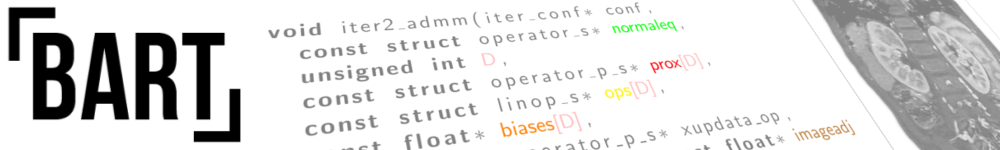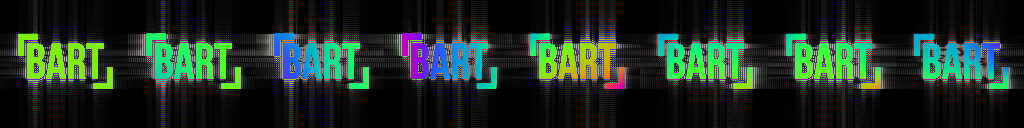# BART: FeaturesFigure: Simulated MRI images.

## List of Features

• Basic features:
• runs on Linux and Mac OS X
• multi-dimensional operations on arrays
• fast non-uniform Fourier Transform (nuFFT and convolution-based method)
• multi-dimensional (divergence-free) wavelet transform
• parallel computation on multiple cores and with Graphical Processing Units (GPU)
• Iterative methods:
• Conjugate Gradients (CG)
• (Fast) Iterative Soft-Thresholding Algorithm (ISTA and FISTA)
• Normalized iterative hard thresholding (NIHT)
• Alternating Direction Method of Multipliers (ADMM)
• Iteratively Regularized Gauss-Newton Method (IRGNM)
• Chambolle-Pock primal-dual algorithm
• Calibration methods:
• direct calibration of coil sensitivities from k-space center
• Walsh's method for calibration of coil sensitivities
• ESPIRiT
• (geometric) channel compression and whitening
• RING: estimation of gradient delays for radial MRI
• reconstruction methods for MRI:
• iterative parallel imaging reconstruction: POCSENSE, SENSE
• compressed sensing and parallel imaging
• calibration-less parallel imaging: NLINV and ENLIVE (non-linear optimization) and SAKE (structured low-rank matrix completion)
• reconstruction with linear subspace constraints
• non-linear model-based reconstruction for T1, T2, flow, and water-fat mapping
• methods based on deep learning: variational networks and MoDL
• Regularization (in arbitrary dimensions):
• Tikhonov
• total variation
• l1-wavelet
• (multi-scale) low-rank
• Tensorflow-based priors